Next: Moments of Distribution Function Up: Plasma Fluid Theory Previous: Plasma Fluid Theory

# Introduction

In plasma fluid theory, a plasma is characterized by a few local parameters--such as the particle density, the kinetic temperature, and the flow velocity--the time evolutions of which are determined by means of fluid equations. These equations are analogous to, but generally more complicated than, the equations of gas dynamics.

Fluid equations are conventionally obtained by taking velocity space moments of the kinetic equation (see Section 3.2),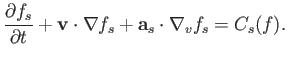(4.1)

Here,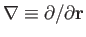,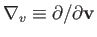, and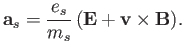(4.2)

Furthermore,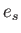and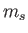are the species-electrical charge and mass, respectively, whereasand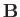are the ensemble-averaged electromagnetic fields.

In general, it is extremely difficult to solve the kinetic equation directly, because of the complexity of the collision operator. However, there are some situations in which collisions can be completely neglected. In such cases, the kinetic equation simplifies to give the so-called Vlasov equation,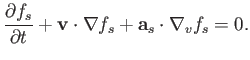(4.3)

The Vlasov equation is tractable in sufficiently simple geometry. (See Chapter 8.) Nevertheless, the fluid approach possesses significant advantages, even in the Vlasov limit. These advantages are as follows.

First, fluid equations involve fewer dimensions than the Vlasov equation. That is, three spatial dimensions instead of six phase-space dimensions. This advantage is especially important in computer simulations.

Second, the fluid description is intuitively appealing. We immediately understand the significance of fluid quantities such as density and temperature, whereas the significance of distribution functions is far less obvious. Moreover, fluid variables are relatively easy to measure in experiments, whereas, in most cases, it is extraordinarily difficult to measure a distribution function accurately. There seems remarkably little point in centering our theoretical description of plasmas on something that we cannot generally measure.

Finally, the kinetic approach to plasma physics is spectacularly inefficient. The species distribution functions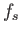provide vastly more information than is needed to obtain the constitutive relations [i.e., Equations (3.1) and (3.2)] that close Maxwell's equations. (See Section 3.2.) After all, these relations only depend on the two lowest moments of the species distribution functions.Next: Moments of Distribution Function Up: Plasma Fluid Theory Previous: Plasma Fluid Theory
Richard Fitzpatrick 2016-01-23